Next: Properties of the Dirac Up: What is Green's Function Previous: PROPERTIES OF THE GF

# Dirac delta function

The Dirac delta function (sometimes called the unit impulse function) is important in the study of phenomena of an impulsive nature. The Green's Function is the impulse response of a differential equation, so the Dirac delta function has a central role in the method of Green's functions.

The Dirac delta function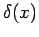is defined to be zero when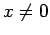, and infinite at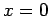in such a way that the area under the function is unity. A concise definition is the following: given non-zero numbers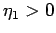and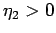,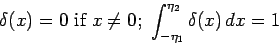ASIDE: This is a weak'' definition of, since the limits of integration are never allowed to be precisely zero. This definition is sufficient for work with Green's functions. See Barton (1989, p. 11) for a discussion of weak'' and strong'' definitions.

Subsections

2004-01-21